【PTA】7-8 到底有多二 (15分)

+关注继续查看

此程序还有一个训练点通不过 希望有大佬能指出问题所在

-13142223336

81.82%

#include <string.h>
#include <stdio.h>
#include <math.h>
int main()
{
double sum=1.0,cd;
int cnt=0,shu=0,len;
char N;
gets(N);
len=strlen(N);
if(N=='-') len-=1;
for(int i=0;i<=len;i++){
if(N[i]=='2') cnt++;
}
if(N=='-') sum*=1.5;
else shu+=N;
for(int j=1;j<=len;j++){
shu=shu*10+N[j];
}
if(shu%2==0) sum*=2;
cd=1.0*cnt/len*sum*100;
printf("%.2lf%%",cd);
return 0;

}10084 0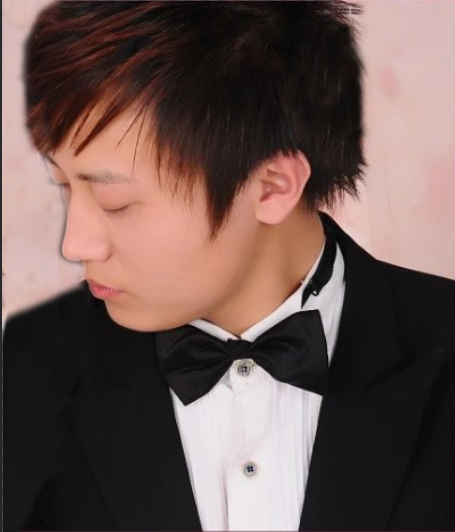6765 014498 0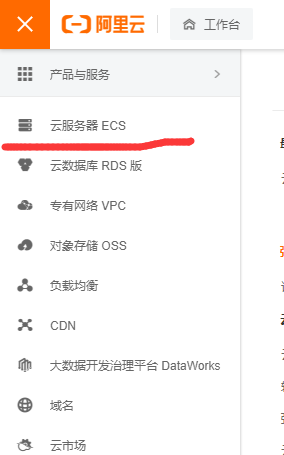2511 0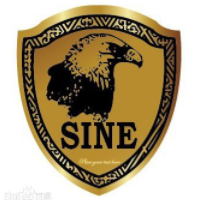windows server 2008阿里云ECS服务器安全设置

9161 013890 07365 0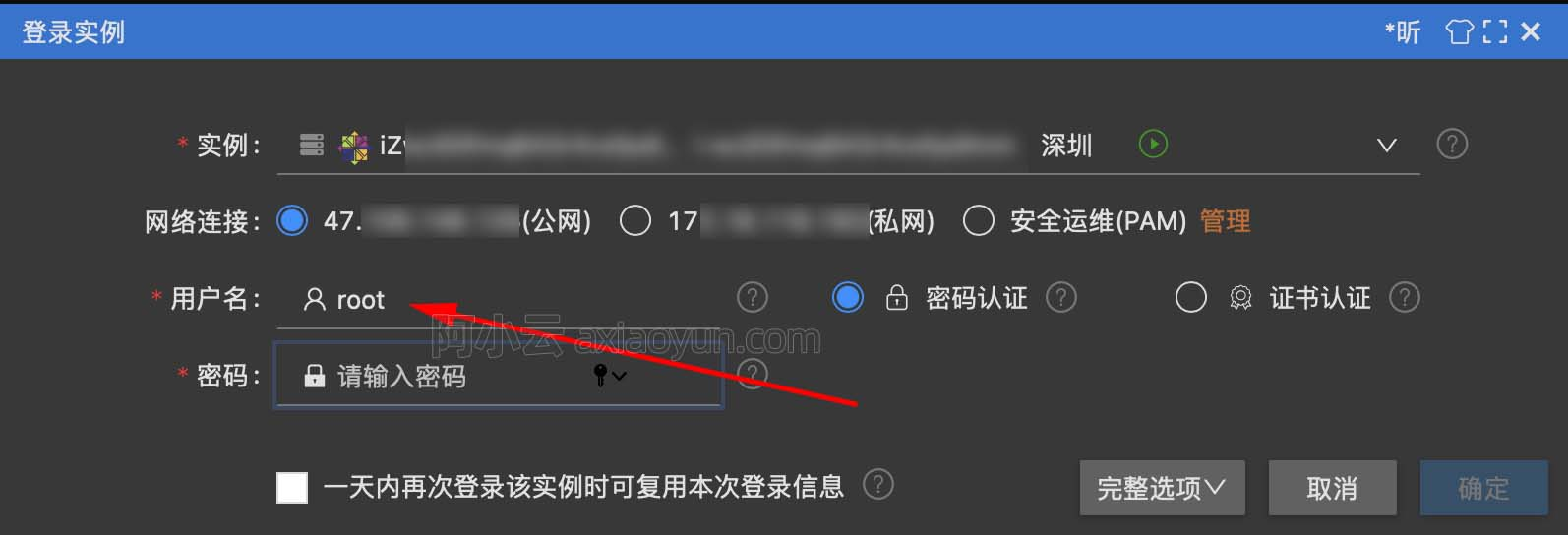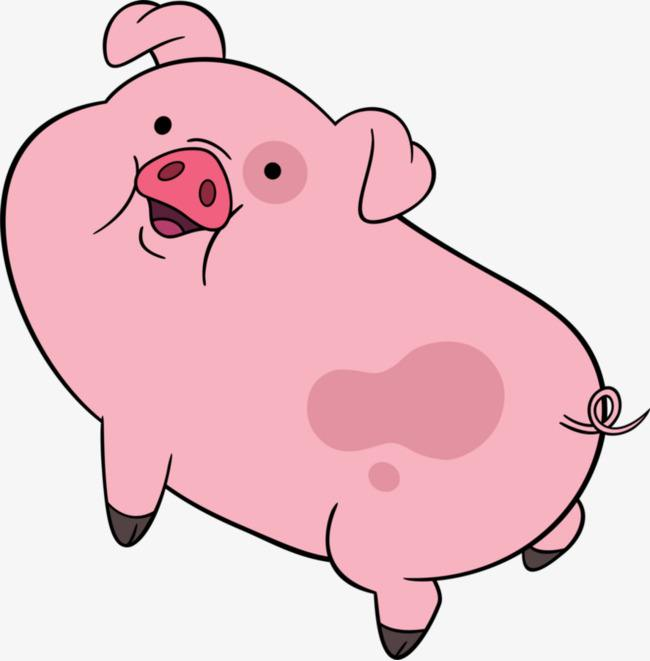4506 022404 054766 0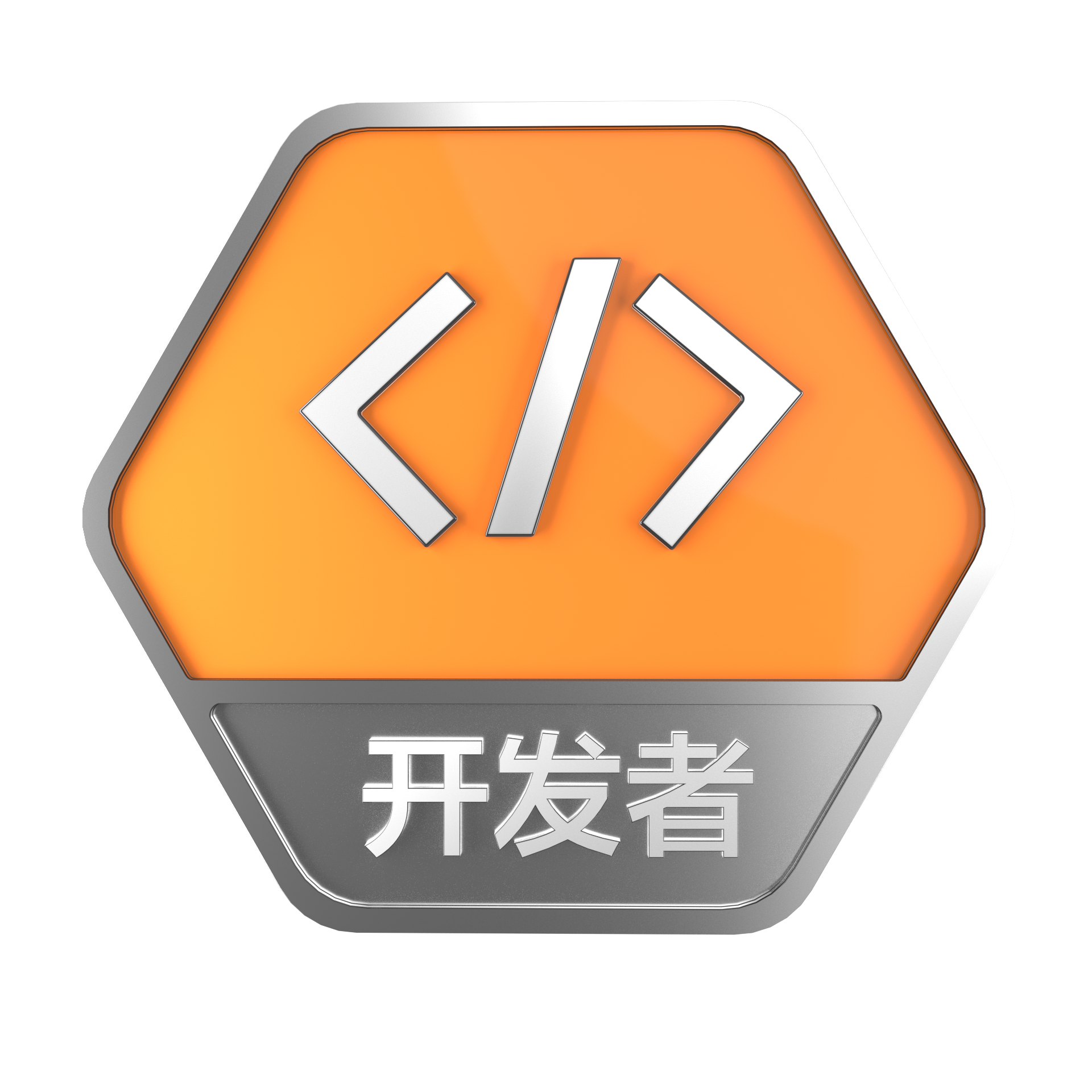+关注
35

0

《2021云上架构与运维峰会演讲合集》

《零基础CSS入门教程》

《零基础HTML入门教程》